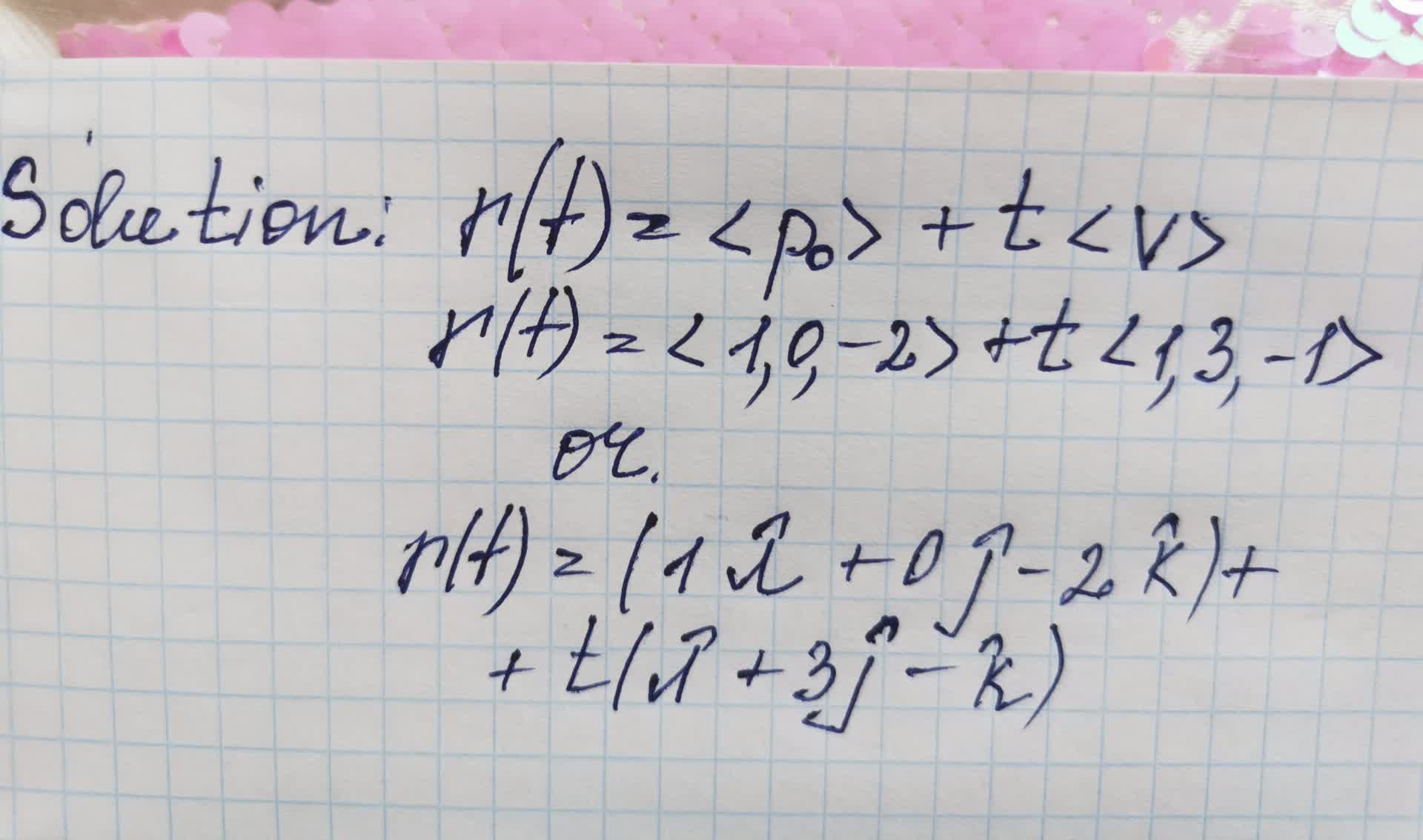Question# Using the point P_{0}=(1,0,-2) and the vector v=(1,3,-1) write a parametric vector e

Parametric equations, polar coordinates, and vector-v
ANSWEREDUsing the point $$\displaystyle{P}_{{{0}}}={\left({1},{0},-{2}\right)}$$ and the vector $$v=(1,3,-1)$$ write a parametric vector equation for a line through $$\displaystyle{P}_{{{0}}}$$ in the direction of v